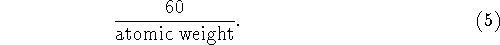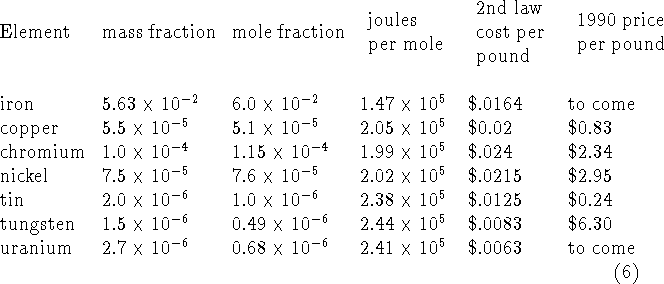Next: About this document Up: The Second Law of Previous: Theory

# Application to Mineral Extraction from ``country rock''

The CRC Handbook of Chemistry and Physics gives the concentrations in milligrams per kilogram of elements in Earth's crust. We chose iron and the five metals of the 1980-1990 Ehrlich-Simon bet.To apply formula (4) we need to use the concentrations by weight given in the Handbook to compute the mole fractions of the ores of the elements in the table in earth's crust. However, we don't know the average molecular weight of the earth's crust. The earth's crust is 57 percent SiO, which has molecular weight 60, so let's take that as the average, although maybe it should be larger. We also don't know what molecules the elements of interest are found in, but the only thing that matters for the purpose of computing their mole fractions is how many atoms of the particular element are in a molecule. We make the more conservative assumption is that there is one atom per molecule, e.g. we are assuming FeO rather than FeO. The mole fraction of the ore of an element can then be obtained by multiplying its mass fraction byWe also must decide on a temperature at which we imagine the extractions to take place. We have chosen 1000 degrees Kelvin as the temperature--for lack of anything better and for the benefit of the arithmetic. Then (4) gives the energy required to extract one mole of the mineral containing the element. We assume that the energy costs \$.05 per kilowatt hour, and this enables us to compare the second law part of the cost of extracting the mineral from random rock with the 1990 price of the element.

These calculations are summarized in the table:Remarks:

• These calculations were done using (1) rather than the approximation. The results aren't much different.
• The costs are proportional to the temperature of the separation. We assumed 1000K, which corresponds to a temperature of smelting. For the real minimum we might have taken 300K, i.e. ambient temperature on the surface of the earth, imagining that a biological process operating at ambient temperature might be devised to do the separation. The costs would then be three times lower.

One might imagine that the cost could be reduced by refrigerating the mixture, performing the separation, and then heating up the results. The second law of thermodynamics is proof against such tricks, so I suppose the specific heat of separated materials would be enough larger than the specific heat of the mixture so that the procedure wouldn't win. It would be a different story if the cooling and the subsequent warming were free because of the existence of heat reservoirs of the desired temperatures, but then we would be getting the energy of separation essentially by running a heat engine between these two temperatures.

• The heavy elements like tungsten and uranium come out with cheaper second law costs than lighter elements, because you get more pounds per mole. The second law costs are proportional to the number of moles, and the prices are per pound.
• The second law energy costs come out trivial compared to the 1990 prices, because they are only a tiny fraction of the real costs of extracting minerals. With very low grade ores they will still be trivial, because the real cost of a unit of mineral is likely to be inversely proportional to the concentration, since the amount of material must be handled is inversely proportional to the concentration. The second law costs per unit of mineral obtained are only proportional to the negative logarithm of the concentration, which grows much more slowly as the mineral becomes rarer.

There are also energy costs associated with breaking the chemical bonds associated the minerals. These are proportional to the amount of mineral extracted provided that, as is often the case, mechanical separation of some kind can be used to concentrate the ore. The Second Law costs discussed in this article are partly included in the ore concentration costs.

In short, the claim that second law costs will do us in is nonsense.

• The one case in which second law costs of separation are probably significant is in the desalination of water. The reason is that fresh water is required for agriculture in quantities much greater than all other substances used by our civilization.Next: About this document Up: The Second Law of Previous: Theory

John McCarthy
Thu Aug 21 09:12:21 PDT 1997